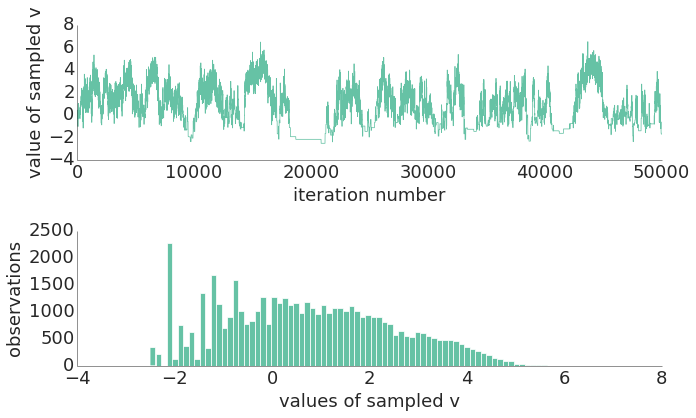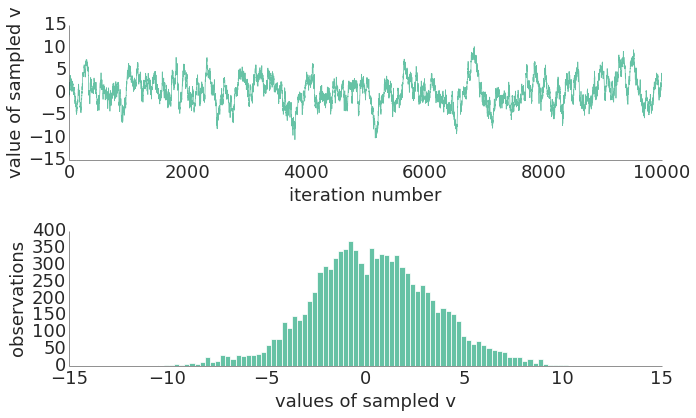### Metropolis-Hastings and slice sampling in Python

One really interesting question from a CS 281 assignment this past semester involved comparing Metropolis-Hastings and slice sampling on a joint distribution. Here is a hierarchical model that looks like a ten-dimensional "funnel":

$$v \sim \mathcal{N}(v|0,3^2)$$$$x_k|v \sim \mathcal{N}(x_k|0,e^v) \text{ for } k=1,2,...,9$$

This model leads to a joint distribution:

$$p(v, x_1, x_2, ..., x_9) = \mathcal{N}(v|0, 3^2) \prod_{k=1}^9 \mathcal{N}(x_k|0,e^v)$$

In this model, we first pick $v$ and then pick our $x_k$s. We can pretty easily write a Python object to model this joint distribution. One way to do that would be to subclass scipy.stats.rv_continuous, but that's sort of overkill for our purposes here (and in my experience can be pretty slow when calling rvs).

In :
from __future__ import division

import numpy as np
import scipy.stats as ss

class joint_dist(object):

def rvs(self, n=1):
""" sample a random variable from this distribution """
sample = np.zeros((10, n))

for i in xrange(n):
# generate rvs
v = ss.norm(0, 3).rvs()
xs = ss.norm(0, np.sqrt(np.e**v)).rvs(9)
# place in sample array
sample[0, i] = v
sample[1:, i] = xs

return sample

def pdf(self, sample):
""" get the probability of a specific sample """
v = sample
pv = ss.norm(0, 3).pdf(v)
xs = sample[1:]
pxs = [ss.norm(0, np.sqrt(np.e**v)).pdf(x_k) for x_k in xs]
return np.array([pv] + pxs)

def loglike(self, sample):
""" log likelihood of a specific sample """
return np.sum(np.log(self.pdf(sample)))


Pretend you don't know about the directed structure and that you just had the joint distribution. Use Metropolis-Hastings to generate 50,000 samples. Use a ten-dimensional Gaussian proposal distribution with identity covariance, centered at the current state. That is, if ${\boldsymbol{w}=[v, x_1, \ldots, x_9]}$, then $q(\boldsymbol{w}'\gets \boldsymbol{w}) = \mathcal{N}(\boldsymbol{w}' | \boldsymbol{w}, \mathbb{I}_{10})$. Start from ${v=0}$ and ${x_k=1}$. Plot a histogram of $v$. How many samples had ${v<-5}$?

## Metropolis-Hastings¶

The snippet below was converted from MATLAB code found in an excellent presentation by Ryan Adams.

In :
def metropolis(init, iters):
"""
based on http://www.cs.toronto.edu/~asamir/cifar/rpa-tutorial.pdf

can take several minutes to run with large sample sizes.
"""
dist = joint_dist()

# set up empty sample holder
D = len(init)
samples = np.zeros((D, iters))

# initialize state and log-likelihood
state = init.copy()
Lp_state = dist.loglike(state)

accepts = 0
for i in np.arange(0, iters):

# propose a new state
prop = np.random.multivariate_normal(state.ravel(), np.eye(10)).reshape(D, 1)

Lp_prop = dist.loglike(prop)
rand = np.random.rand()
if np.log(rand) < (Lp_prop - Lp_state):
accepts += 1
state = prop.copy()
Lp_state = Lp_prop

samples[:, i] = state.copy().ravel()

# if i % 1000 == 0: print i

print 'Acceptance ratio', accepts/iters
return samples


Let's start by taking 50,000 samples using Metropolis-Hastings.

In :
import prettyplotlib as pplt

from matplotlib import rcParams
rcParams['font.size'] = 18
rcParams['figure.figsize'] = (10, 6)

# define our starting point
w_0 = np.array([0., 1., 1., 1., 1., 1., 1., 1., 1., 1.])

# actually do the sampling
n = 50000
samples = metropolis(w_0, n)
v = samples[0, :]

fig, (ax0, ax1) = plt.subplots(2, 1)

# show values of sampled v by iteration
pplt.plot(ax0, np.arange(n), v)
ax0.set_xlabel('iteration number')
ax0.set_ylabel('value of sampled v')

# plot a histogram of values of v
pplt.hist(ax1, v, bins=80)
ax1.set_xlabel('values of sampled v')
ax1.set_ylabel('observations')

plt.tight_layout()

Acceptance ratio 0.20544As for how many samples have $v < -5$:

In :
v[v < -5].size

Out:
0

Not a single sample. Looking at the implementation above, it's easy to reason out why this happened. The log-likelihood is measuring the non-naive probability (that is, it assumes that $v$ was chosen first and that each $x_k$'s variance actually corresponds to $v$). That in turn means that — under the directed regime — any small or negative $v$ implies that every $x_k \sim \mathcal{N}(0,\; e^v \approx 0)$, thus imposing a huge probability "penalty" on any non-zero $x_k$. Meanwhile, our Metropolis-Hastings is naively proposing a vector of $x_k$s which are probably not all zero, so we tend to reject any small or negative $v$.

We can see that pretending order doesn't matter is a bad idea here! Instead of ending up with a symmetrical, Gaussian-looking distribution for $v$, it is badly skewed.

## Slice sampling¶

One improvement in this case would be to use slice sampling.

Implement slice sampling on the same joint distribution. Use any variant of slice sampling you wish (e.g., coordinate-wise, random directions, hyperrectangle, exponential step-out, etc.). Specify which you use. Plot a histogram of $v$. How many samples had ${v< -5}$?

This particular Python implementation of coordinate-wise slice sampling is adapted from MATLAB code found on Iain Murray's site.

In :
def slice_sample(init, iters, sigma, step_out=True):
"""
based on http://homepages.inf.ed.ac.uk/imurray2/teaching/09mlss/
"""

dist = joint_dist()

# set up empty sample holder
D = len(init)
samples = np.zeros((D, iters))

# initialize
xx = init.copy()

for i in xrange(iters):
perm = range(D)
np.random.shuffle(perm)
last_llh = dist.loglike(xx)

for d in perm:
llh0 = last_llh + np.log(np.random.rand())
rr = np.random.rand(1)
x_l = xx.copy()
x_l[d] = x_l[d] - rr * sigma[d]
x_r = xx.copy()
x_r[d] = x_r[d] + (1 - rr) * sigma[d]

if step_out:
llh_l = dist.loglike(x_l)
while llh_l > llh0:
x_l[d] = x_l[d] - sigma[d]
llh_l = dist.loglike(x_l)
llh_r = dist.loglike(x_r)
while llh_r > llh0:
x_r[d] = x_r[d] + sigma[d]
llh_r = dist.loglike(x_r)

x_cur = xx.copy()
while True:
xd = np.random.rand() * (x_r[d] - x_l[d]) + x_l[d]
x_cur[d] = xd.copy()
last_llh = dist.loglike(x_cur)
if last_llh > llh0:
xx[d] = xd.copy()
break
elif xd > xx[d]:
x_r[d] = xd
elif xd < xx[d]:
x_l[d] = xd
else:
raise RuntimeError('Slice sampler shrank too far.')

if i % 1000 == 0: print 'iteration', i

samples[:, i] = xx.copy().ravel()

return samples


Let's do the sampling and plotting again using slice sampling instead of Metropolis-Hastings. This time, we'll only do 10,000 iterations because it takes longer — much longer, actually.

In :
# define our starting point
w_0 = np.array([0., 1., 1., 1., 1., 1., 1., 1., 1., 1.])

# actually do the sampling
n = 10000
sigma = np.ones(10)
samples = slice_sample(w_0, iters=n, sigma=sigma)
v = samples[0, :]

fig, (ax0, ax1) = plt.subplots(2, 1)

# show values of sampled v by iteration
pplt.plot(ax0, np.arange(n), v)
ax0.set_xlabel('iteration number')
ax0.set_ylabel('value of sampled v')

# plot a histogram of values of v
pplt.hist(ax1, v, bins=80)
ax1.set_xlabel('values of sampled v')
ax1.set_ylabel('observations')

plt.tight_layout()

iteration 0
iteration 1000
iteration 2000
iteration 3000
iteration 4000
iteration 5000
iteration 6000
iteration 7000
iteration 8000
iteration 9000In :
v[v < -5].size

Out:
377

Here, we actually do see small and negative values for $v$ as we expected, and the resulting distribution looks much more Gaussian. (Note that for both types of sampling, we could have discarded a certain number of samples for "burn in," but it's interesting to see the entire arc of how the sampling panned out.)

Any comments or suggestions? Let me know.